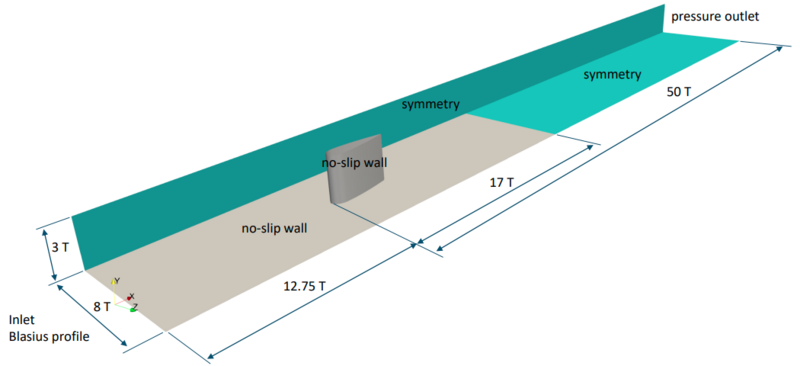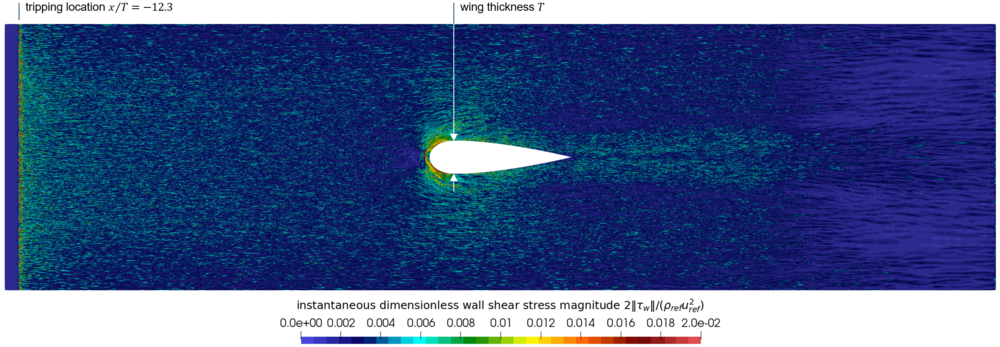# Introduction

This test case considers the flow around a wing mounted on a flat plate, which is representative of the wing-body junction flow problems encountered in applications of aeronautical interest. The flow features the interaction between the incipient turbulent boundary layer and the mounted airfoil, and the main physical phenomenon of interest is the horseshoe vortex developing at the junction and the corner separation. This flow is also highly 3D and anisotropic regarding the turbulent stresses. Establishing a DNS database of this flow is of crucial interest since it has been shown that RANS models (both Boussinesq and Reynolds stresses-based models) display strong difficulties in recovering data from the available experiments. Such a database allows for a more thorough availability of the flow field with respect to the experiments and gives the possibility of using Machine Learning or data-assimilation techniques to improve standard RANS models.

# Review of previous studies and choice of test case

A thorough listing of existing experimental and numerical studies regarding wing-body junction flows can be found in Gand et al. (2010). The present uDNS is based on the configuration considered in the simulations by Apsley and Leschziner (2001), who were based themselves on the experimental studies by Devenport and Simpson (1990) and Fleming et al. (1995). The Reynolds number based on the airfoil thickness is similar to the experiment and its value is $115,000$. The flow is almost incompressible with a Mach number based on the freestream velocity of $0.078$. The uDNS setup reproduces the experimental conditions but with half the experimental impacting boundary layer thickness. Therefore a direct comparison with the experimental results is not possible for the present uDNS.

# Description of the test case

## Geometry and flow parameters

Fig. 2 displays the flow geometry, i.e. the wing body mounted on a flat plate, and the computional domain. The wing is formed by a 3:2 semi-elliptic nose with a NACA0020 tail profile, see Fig. 3, and has a thickness $T=71.7\,{\text{mm}}$, which serves as reference lengthscale. The Reynolds number based on this is $Re=115\,000$and the chord-to-thickness ratio is $4.254$. The computational domain size is $62.75T$in the streamwise direction, $8T$in the spanwise direction and $3T$in the wall-normal direction, see Fig. 2. The coordinate origin is located at the root leading edge of the airfoil. There is no flow incidence relatively to the wing, corresponding to an angle of attack of $0$degrees. Parameters of the flow (air with $\gamma =1.4$, $MM=28.96\,{\text{kg/kmol}}$, $\mu =1.716\cdot 10^{-5}\,{\text{Pa s}}$) are reported in Tab. 1.Figure 2: Wing-body junction. Flow geometry and domain for the DNS simulationFigure 3: Wing-body junction. Wing geometry, thickness and location of boundary layer tripping superimposed by the instantaneous wall shear stress on the flat plate

 ${Ma}$${Re}$${T_{ref}}$${u_{ref}}$${\rho _{ref}}$${p_{ref}}$${0.078}$${115\,000}$${298.15\,{\text{K}}}$${27.00\,{\text{m/s}}}$${1.019\,{\text{kg/m}}^{3}}$${87251\,{\text{Pa}}}$Table 1: Wing-body junction. Flow parameters

## Boundary conditions

The computational set-up aims at replicating the flow conditions of the experiment but with a halved incoming boundary layer thickness. To enforce these conditions in an efficient and accurate manner a Blasius velocity profile corresponding to $Re_{x}=900\,000$and free stream velocity $u_{\infty }=u_{ref}$is imposed at the inlet together with uniform static pressure $p_{inflow}=p_{ref}$. and total temperature $T_{t,inflow}=T_{ref}\left[1+0.5\left(\gamma -1\right){Ma}^{2}\right]$. A laminar boundary layer is then established, and a flow perturbation is applied at $Re_{x}=950\,000$to trigger transition to turbulence following the idea of Housseini et al. (2016) and Schlatter and Örlü (2012). The distance of the inlet boundary from the wing-body junction is defined such that the incoming turbulent boundary layer reaches a thickness $\delta _{99.5}/T=0.25$(half of the experimental value) at a given point, hereinafter referred as checkpoint, upstream the airfoil leading edge, i.e., at $x_{ckp}/T=-2.15$. To define such distance, a precursory computational campaign for the turbulent flow over a flat plate was performed. According to the outcomes of this campaign, the inlet boundary is positioned at $x/T=-12.75$. The tripping term promoting the boundary layer transition is then located at $x/T=-12.3$, see Fig. 3. At the outlet boundary, placed at $x/T=50$, the static pressure ${p_{outflow}=p_{ref}}$is imposed with an exit-pressure outflow boundary condition. The bottom boundary is an adiabatic solid wall type (no slip) for $-12.75\leq x/T\leq 17$and symmetry type for $x/T>17$. This choice aims to mitigate spurious perturbations possibly originating at the outlet boundary. Symmetry conditions are imposed at the lateral ($z/T=\pm 4$) and top ($y/T=3$) boundaries. An adiabatic solid wall(no slip) condition is finally imposed on the wing surface.